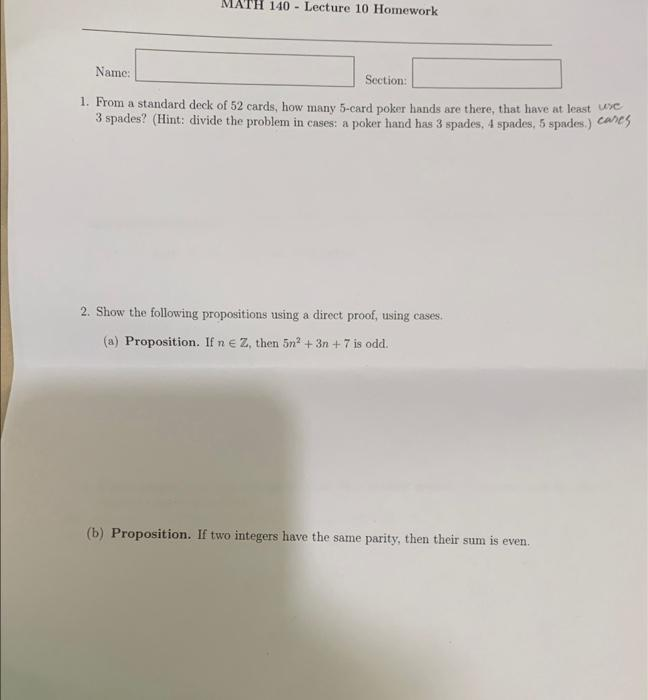Home / Expert Answers / Other Math / name-math-140-lecture-10-homework-section-1-from-a-standard-deck-of-52-cards-how-many-5-card-p-pa240

# (Solved): Name: MATH 140 - Lecture 10 Homework Section: 1. From a standard deck of 52 cards, how many 5-card p ...

Name: MATH 140 - Lecture 10 Homework Section: 1. From a standard deck of 52 cards, how many 5-card poker hands are there, that have at least use 3 spades? (Hint: divide the problem in cases: a poker hand has 3 spades, 4 spades, 5 spades.) cares 2. Show the following propositions using a direct proof, using cases. (a) Proposition. If n E Z, then 5n² + 3n +7 is odd. (b) Proposition. If two integers have the same parity, then their sum is even.1. From a standard deck of 52 cards, how many 5 -card poker hands are there, that have at least wse 3 spades? (Hint: divide the problem in cases: a poker hand has 3 spades, 4 spades, 5 spades.) cares 2. Show the following propositions using a direct proof, using cases. (a) Proposition. If , then is odd. (b) Proposition. If two integers have the same parity, then their sum is even.

We have an Answer from Expert